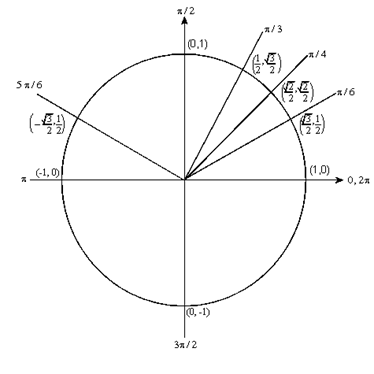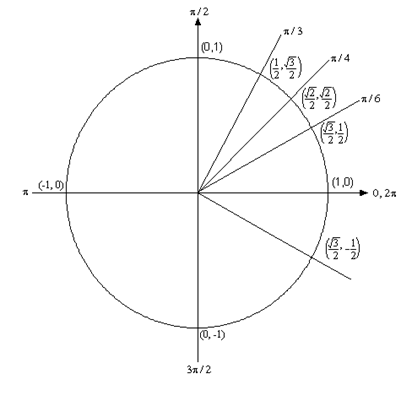Paul's Online Notes
Home / Algebra Trig Review / Trigonometry / Inverse Trig Functions
Show Mobile Notice Show All Notes Hide All Notes
Mobile Notice
You appear to be on a device with a "narrow" screen width (i.e. you are probably on a mobile phone). Due to the nature of the mathematics on this site it is best views in landscape mode. If your device is not in landscape mode many of the equations will run off the side of your device (should be able to scroll to see them) and some of the menu items will be cut off due to the narrow screen width.

### Inverse Trig Functions

One of the more common notations for inverse trig functions can be very confusing. First, regardless of how you are used to dealing with exponentiation we tend to denote an inverse trig function with an “exponent” of “-1”. In other words, the inverse cosine is denoted as $${\cos ^{ - 1}}\left( x \right)$$. It is important here to note that in this case the “-1” is NOT an exponent and so,

${\cos ^{ - 1}}\left( x \right) \ne \frac{1}{{\cos \left( x \right)}}$

In inverse trig functions the “-1” looks like an exponent but it isn’t, it is simply a notation that we use to denote the fact that we’re dealing with an inverse trig function. It is a notation that we use in this case to denote inverse trig functions. If I had really wanted exponentiation to denote 1 over cosine I would use the following.

${\left( {\cos \left( x \right)} \right)^{ - 1}} = \frac{1}{{\cos \left( x \right)}}$

There’s another notation for inverse trig functions that avoids this ambiguity. It is the following.

\begin{align*}{\cos ^{ - 1}}\left( x \right) & = \arccos \left( x \right)\\ {\sin ^{ - 1}}\left( x \right) & = \arcsin \left( x \right)\\ {\tan ^{ - 1}}\left( x \right) & = \arctan \left( x \right)\end{align*}

So, be careful with the notation for inverse trig functions!

There are, of course, similar inverse functions for the remaining three trig functions, but these are the main three that you’ll see in a calculus class so I’m going to concentrate on them.

To evaluate inverse trig functions remember that the following statements are equivalent.

\begin{align*}\theta & = {\cos ^{ - 1}}\left( x \right) & \hspace{0.5in} & \Leftrightarrow & \hspace{0.5in} x & = \cos \left( \theta \right)\\ \theta & = {\sin ^{ - 1}}\left( x \right) & \hspace{0.5in} & \Leftrightarrow & \hspace{0.5in} x & = \sin \left( \theta \right)\\ \theta & = {\tan ^{ - 1}}\left( x \right) & \hspace{0.5in} & \Leftrightarrow & \hspace{0.5in} x & = \tan \left( \theta \right)\end{align*}

In other words, when we evaluate an inverse trig function we are asking what angle, $$\theta$$, did we plug into the trig function (regular, not inverse!) to get $$x$$.

So, let’s do some problems to see how these work. Evaluate each of the following. Show All Solutions Hide All Solutions

1. $$\displaystyle {\cos ^{ - 1}}\left( {\frac{{\sqrt 3 }}{2}} \right)$$
Show Solution

In Problem 1 of the Solving Trig Equations section we solved the following equation.

$\cos \left( t \right) = \frac{{\sqrt 3 }}{2}$

In other words, we asked what angles, $$x$$, do we need to plug into cosine to get $$\frac{{\sqrt 3 }}{2}$$? This is essentially what we are asking here when we are asked to compute the inverse trig function.

${\cos ^{ - 1}}\left( {\frac{{\sqrt 3 }}{2}} \right)$

There is one very large difference however. In Problem 1 we were solving an equation which yielded an infinite number of solutions. These were,

\begin{align*} & \frac{\pi }{6} + 2\pi \,n\,,\;\;n = 0,\, \pm 1,\, \pm 2,\, \pm 3,\, \ldots \\ & \frac{{11\pi }}{6} + 2\pi \,n\,,\;\;n = 0,\, \pm 1,\, \pm 2,\, \pm 3,\, \ldots \end{align*}

In the case of inverse trig functions, we are after a single value. We don’t want to have to guess at which one of the infinite possible answers we want. So, to make sure we get a single value out of the inverse trig cosine function we use the following restrictions on inverse cosine.

$\theta = {\cos ^{ - 1}}\left( x \right)\hspace{0.25in}\hspace{0.25in} - 1 \le x \le 1\hspace{0.25in}{\rm{and }}\hspace{0.25in}0 \le \theta \le \pi$

The restriction on the $$\theta$$ guarantees that we will only get a single value angle and since we can’t get values of $$x$$ out of cosine that are larger than 1 or smaller than -1 we also can’t plug these values into an inverse trig function.

So, using these restrictions on the solution to Problem 1 we can see that the answer in this case is

${\cos ^{ - 1}}\left( {\frac{{\sqrt 3 }}{2}} \right) = \frac{\pi }{6}$
2. $$\displaystyle {\cos ^{ - 1}}\left( { - \frac{{\sqrt 3 }}{2}} \right)$$
Show Solution

In general, we don’t need to actually solve an equation to determine the value of an inverse trig function. All we need to do is look at a unit circle. So, in this case we’re after an angle between 0 and $$\pi$$ for which cosine will take on the value $$- \frac{{\sqrt 3 }}{2}$$. So, check out the following unit circleFrom this we can see that

${\cos ^{ - 1}}\left( { - \frac{{\sqrt 3 }}{2}} \right) = \frac{{5\pi }}{6}$
3. $$\displaystyle {\sin ^{ - 1}}\left( { - \frac{1}{2}} \right)$$
Show Solution

The restrictions that we put on $$\theta$$ for the inverse cosine function will not work for the inverse sine function. Just look at the unit circle above and you will see that between 0 and $$\pi$$ there are in fact two angles for which sine would be $$\frac{1}{2}$$ and this is not what we want. As with the inverse cosine function we only want a single value. Therefore, for the inverse sine function we use the following restrictions.

$\theta = {\sin ^{ - 1}}\left( x \right)\hspace{0.25in}\hspace{0.25in} - 1 \le x \le 1\hspace{0.25in}{\rm{and }}\hspace{0.25in} - \frac{\pi }{2} \le \theta \le \frac{\pi }{2}$

By checking out the unit circlewe see

${\sin ^{ - 1}}\left( { - \frac{1}{2}} \right) = - \frac{\pi }{6}$
4. $${\tan ^{ - 1}}\left( 1 \right)$$
Show Solution

The restriction for inverse tangent is

$\theta = {\tan ^{ - 1}}\left( x \right)\hspace{0.25in}\hspace{0.25in}\hspace{0.25in} - \frac{\pi }{2} < \theta < \frac{\pi }{2}$

Notice that there is no restriction on $$x$$ this time. This is because $$\tan \left( \theta \right)$$can take any value from negative infinity to positive infinity. If this is true then we can also plug any value into the inverse tangent function. Also note that we don’t include the two endpoints on the restriction on $$\theta$$. Tangent is not defined at these two points, so we can’t plug them into the inverse tangent function.

In this problem we’re looking for the angle between $$- \frac{\pi }{2}$$ and $$\frac{\pi }{2}$$ for which $$\tan \left( \theta \right) = 1$$, or $$\sin \left( \theta \right) = \cos \left( \theta \right)$$. This can only occur at $$\theta = \frac{\pi }{4}$$ so,

${\tan ^{ - 1}}\left( 1 \right) = \frac{\pi }{4}$
5. $$\displaystyle \cos \left( {{{\cos }^{ - 1}}\left( {\frac{{\sqrt 3 }}{2}} \right)} \right)$$
Show Solution

Recalling the answer to Problem 1 in this section the solution to this problem is much easier than it looks like on the surface.

$\cos \left( {{{\cos }^{ - 1}}\left( {\frac{{\sqrt 3 }}{2}} \right)} \right) = \cos \left( {\frac{\pi }{6}} \right) = \frac{{\sqrt 3 }}{2}$

This problem leads to a couple of nice facts about inverse cosine

$\cos \left( {{{\cos }^{ - 1}}\left( x \right)} \right) = x\hspace{0.25in}\hspace{0.25in}{\rm{AND}}\hspace{0.25in}\hspace{0.25in}{\cos ^{ - 1}}\left( {\cos \left( \theta \right)} \right) = \theta$
6. $$\displaystyle {\sin ^{ - 1}}\left( {\sin \left( {\frac{\pi }{4}} \right)} \right)$$
Show Solution

This problem is also not too difficult (hopefully…).

${\sin ^{ - 1}}\left( {\sin \left( {\frac{\pi }{4}} \right)} \right) = {\sin ^{ - 1}}\left( {\frac{{\sqrt 2 }}{2}} \right) = \frac{\pi }{4}$

As with inverse cosine we also have the following facts about inverse sine.

$\sin \left( {{{\sin }^{ - 1}}\left( x \right)} \right) = x\hspace{0.25in}\hspace{0.25in}{\rm{AND}}\hspace{0.25in}\hspace{0.25in}{\sin ^{ - 1}}\left( {\sin \left( \theta \right)} \right) = \theta$
7. $$\tan \left( {{{\tan }^{ - 1}}\left( { - 4} \right)} \right)$$
Show Solution

Just as inverse cosine and inverse sine had a couple of nice facts about them so does inverse tangent. Here is the fact

$\tan \left( {{{\tan }^{ - 1}}\left( x \right)} \right) = x\hspace{0.25in}\hspace{0.25in}{\rm{AND}}\hspace{0.25in}\hspace{0.25in}{\tan ^{ - 1}}\left( {\tan \left( \theta \right)} \right) = \theta$

Using this fact makes this a very easy problem as I couldn’t do $${\tan ^{ - 1}}\left( 4 \right)$$ by hand! A calculator could easily do it, but I couldn’t get an exact answer from a unit circle.

$\tan \left( {{{\tan }^{ - 1}}\left( { - 4} \right)} \right) = - 4$